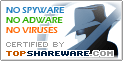# 3DMath Explorer 3.1

SNAPSHOT

3DMath Explorer 3D Graph Plotting Software

Windows AllPlatform :
USD \$45Price :
2.33 MBFile Size :
ScreenshotScreenshot :Popularity :3DMath Explorer is a computer program that pilots 2D and 3D graphs of mathematical functions and curves in unlimited graphing space. It has many useful feature such as 3D curve ploting in real time, perspective drawing, graph scaling (zooming), active graph rotation, fogging effect, cubic draw, unlimited space ploting, four view plot screen, auto rotate animation, drawing many curves in the same screen,working with many graph screen est.

• Linear Algebra
Computations associated with matrices
• Inverse Matrices
Mathematical program for university students.
• Analysis Studio
An intuitive and powerful statistical analysis, regression analysis and data mining tool. Analysis Studio features a fast deep logistic regression model development, deployment and regression analysis
• CrossGL Surface Calculator
• MathRapid
"MathRapid" is an easy brain training game.
• Gigaply
Proper replacement for Windows calculator
• ZigZag
ZigZag is a mathematical graphing tool
• ExS
A free scientific calculator easy enough for anybody to use.
• QuikCalc Mortgage & Loan Manager Personal Edition
Powerful mortgage and loan manager
• Develve
Develve Statistical Software Quality Improvement DOE weibull Gauge R&R samplsize